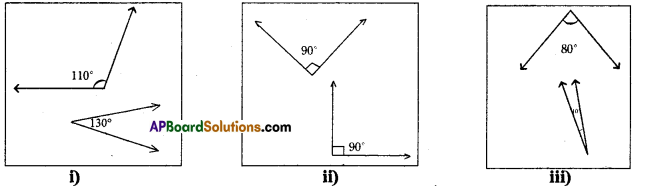AP State Syllabus AP Board 7th Class Maths Solutions Chapter 4 Lines and Angles Ex 2 Textbook Questions and Answers.

## AP State Syllabus 7th Class Maths Solutions 4th Lesson Lines and Angles Exercise 2Question 1.
Which of the following pairs of angles are complementary?Solution:
In fig (i) 110° – 30° = 140° ≠ 90. Hence they are not complementary.
In fig (ii) 90° + 90° = 180 ≠ 90°. Hence they are not complementary.
In fig (iii) 80° + 10° = 90° = 90. Hence they are complementary.

Question 2.
Find the complements of the given angles. Find the complementary angles of the following.
(i) 25°
(ii) 40°
(iii) 89°
(iv) 55°
Solution:
i) Complementary angle of 25° = 90° – 25° = 65°
ii) Complementary angle of 40° = 90° – 40° = 50°
ill) Complementary angle of 89° = 90° – 89° = 1°
iv) Complementary angle of 55° = 90° – 55 = 35°Question 3.
Two angles are complement to each other and are also equal. Find them.
Solution:
Let the angle be x°
Then Its complement = (900_ x°)
By problem, x° = 90° – x°
x° + x°= 90°
2x = 90°
x = $$\frac{90^{\circ}}{2}$$
x = 45°

Question 4.
Manasa says, “Each angle in any pair of complementary angles is always acute”. Do
Solution:
Yes. Each angle ¡n any pair of complementary angles is always acute.
As sum of two angles is 90°, each of the angle must be less than 90°.